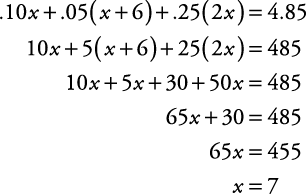## Coin Problems

Here are some examples for calculating money in word problems.

##### Example 1

Tamar has four more quarters than dimes. If he has a total of \$1.70, how many quarters and dimes does he have?

First, circle what you must find— how many quarters and dimes. Let x stand for the number of dimes, then x + 4 is the number of quarters. Therefore, .10 x is the total value of the dimes, and .25( x + 4) is the total value of the quarters. Setting up the following chart can be helpful.

number

value

amount of money

dimes

x

.10

.10x

quarters

x + 4

.25

.25(x + 4)

Now, use the table and problem to set up an equation.So, there are two dimes. Since there are four more quarters, there must be six quarters.

##### Example 2

Sid has \$4.85 in coins. If he has six more nickels than dimes and twice as many quarters as dimes, how many coins of each type does he have?

First, circle what you must find— the number of coins of each type. Let x stand for the number of dimes. Then x + 6 is the number of nickels, and 2 x is the number of quarters. Setting up the following chart can be helpful.

number

value

amount of money

dimes

x

.10

.10x

nickels

x + 6

.05

.05(x + 6)

quarters

2x

.25

.25(2x)

Now, use the table and problem to set up an equation.So, there are seven dimes. Therefore, there are thirteen nickels and fourteen quarters.